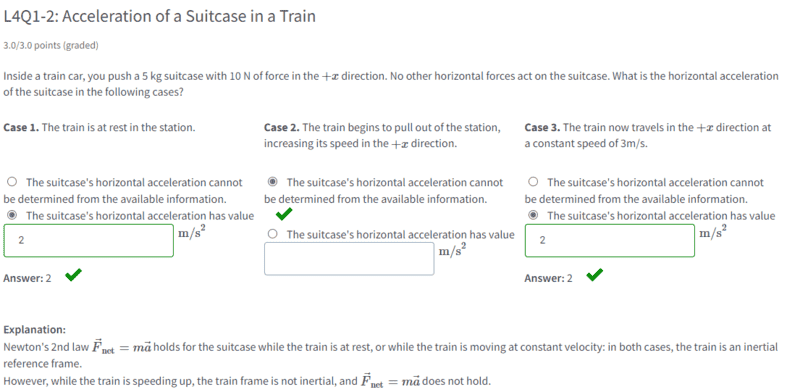# Acceleration of a suitcase in an accelerating train after getting pushed

• String theory guy
String theory guy
Homework Statement
Statement is below.
Relevant Equations
Newton's second law.
I was doing one of MIT's 8.01.1x course and came across this question.In case 2, how would we be able to theoretically calculate the horizontal acceleration in this non-inertial frame? The course said that Newton's Law do not hold in accelerating frames.

However, could we find the acceleration if we knew the friction force from the train floor on the suit case then sum the forces in the x-direction?

Homework Helper
Friction is ignored in this exercise. You may assume the case is on ideal, frictionless wheels.

In the earth frame (considered not accelerating) the only horizontal force acting on the suitcase is the 10 N from your pushing, so the case accelerates to the tune of 2 m/s2.

With respect to the train the acceleration is a sum. Suppose you push forward and the acceleration ##a## of the train is known (e.g. 1 m/s2, also forward), the acceleration of the suitcase wrt the train is ##2-a## m/s2.##\qquad## !​

##\ ##

•String theory guy
Homework Helper
Gold Member
2022 Award
The question is poorly worded. It is entirely unclear which frame of reference you are supposed to use.

••jbriggs444 and String theory guy
Homework Helper
The fact that the suitcase is in the train does not imply that the analysis is (or has) to be done in the reference frame of the train. The suitcase is not in a specific frame depending on the train's motion. You can analyze the motion of the suitcase in the ground frame (and use Newton's law) no matter what the train does.

And here, as they say that there are no other forces acting on the suitcase, the suitcase will not even follow the motion of the train when it (the train) accelerates. It can be as well a suitcase on the platform and a train passing by. The train is irrelevant to the suitcase. At least until it hits the end of the car.

•String theory guy
Homework Helper
Gold Member
2022 Award
The fact that the suitcase is in the train does not imply that the analysis is (or has) to be done in the reference frame of the train
As I noted, it is unclear which reference frame the question assumes. To get the answer marked correct for case 2, it has to be the train's frame.

•String theory guy
Homework Helper
Indeed, the answer make sense with this assumption. But the question should make sense before you know the "right" answer. It is not easy to make good questions, I agree.

••String theory guy and jbriggs444